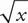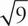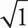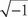# SAT Math Multiple Choice Question 120: Answer and Explanation

### Test Information

Question: 120

15. If 2= x - 3, which of the following is the solution set for x ?

• A. {-1, 9}
• B. {1, -9}
• C. {9}
• D. {1, 9}

C Plug In the Answers! The answers aren't in order, and some numbers appear more than once, so you don't need to start in the middle. Instead, start with 9 because it is in three of the four choices. If x = 9, then 2= 9 - 3.= 3, so the left side of the equation is 2 × 3 = 6, and the right side of the equation is 9 - 3 = 6. This works, so 9 is part of the solution set; eliminate (B) because it doesn't include 9. Next, try x = 1: 2= 1 - 3, which solves to 2 = -2. This isn't true, so 1 is not part of the solution set; eliminate (D). Lastly, try x = -1: 2= -1 - 3. You cannot take the square root of a negative number, so this doesn't work. Eliminate (A) and choose (C).